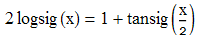# 【深度学习】神经网络入门（最通俗的理解神经网络）

form:http://tieba.baidu.com/p/3013551686?pid=49703036815&see_lz=1#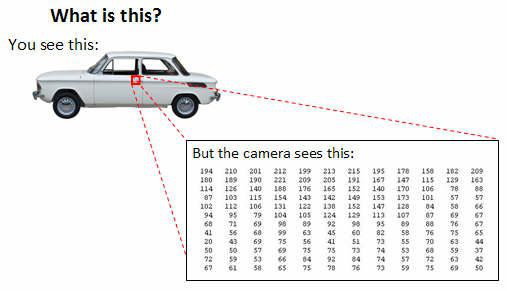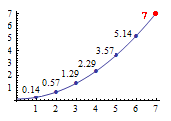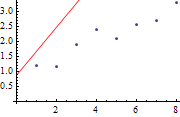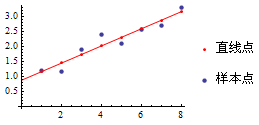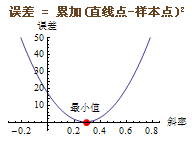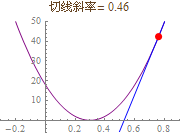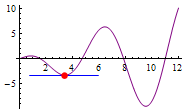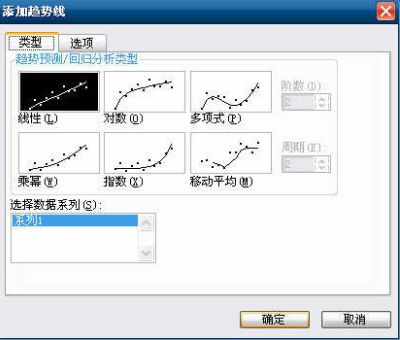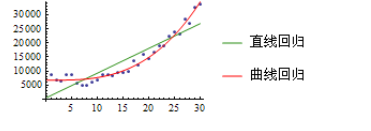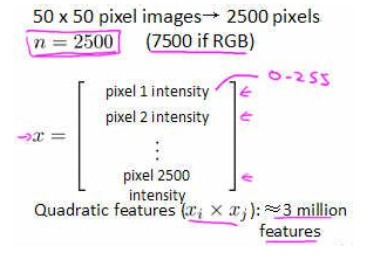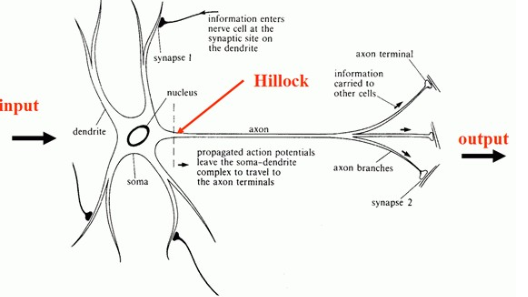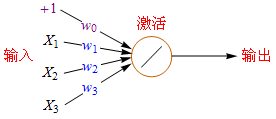X1*w1+X2*w2+…+Xn*wn这种计算方法称为加权求和(Weighted Sum)法，此方法在线性代数里极为常用。加权求和的标准数学符号是，不过为了简化，我在教程里使用女巫布莱尔的符号表示，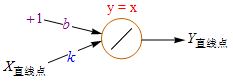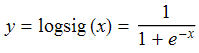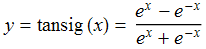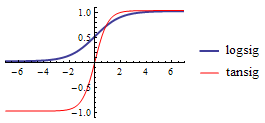e是怎么来的？e = 1 + 1/1! + 1/2! + 1/3! + 1/4! + 1/5! + 1/6! + 1/7! + … = 1 + 1 + 1/2 + 1/6 + 1/24 + 1/120+ … ≈ 2.71828 (!代表阶乘，3!=1*2*3=6)

e在微积分领域非常重要，e^x的导数依然是e^x，自己的导数恰好是它自己，这种巧合在实数范围内绝无仅有。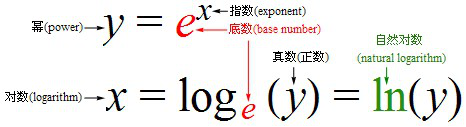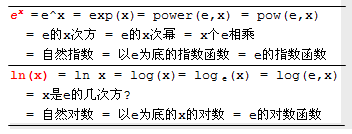e^x和e^-x的图形是对称的；ln(x)是e^x的逆函数，它们呈45度对称。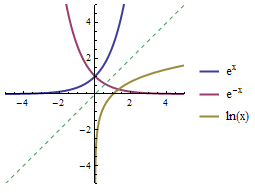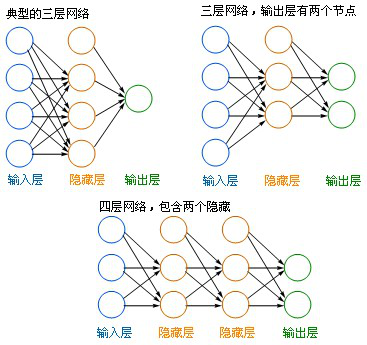• 左边蓝色的圆圈叫“输入层”，中间橙色的不管有多少层都叫“隐藏层”，右边绿色的是“输出层”。
• 每个圆圈，都代表一个神经元，也叫节点(Node)。
• 输出层可以有多个节点，多节点输出常常用于分类问题。
• 理论证明，任何多层网络可以用三层网络近似地表示。
• 一般凭经验来确定隐藏层到底应该有多少个节点，在测试的过程中也可以不断调整节点数以取得最佳效果。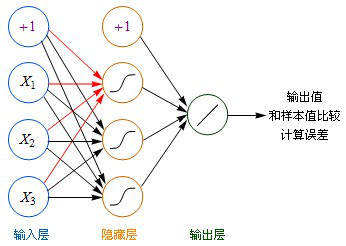• 虽然图中未标识，但必须注意每一个箭头指向的连线上，都要有一个权重(缩放)值。
• 输入层的每个节点，都要与的隐藏层每个节点做点对点的计算，计算的方法是加权求和+激活，前面已经介绍过了。(图中的红色箭头指示出某个节点的运算关系)
• 利用隐藏层计算出的每个值，再用相同的方法，和输出层进行计算。
• 隐藏层用都是用Sigmoid作激活函数，而输出层用的是Purelin。这是因为Purelin可以保持之前任意范围的数值缩放，便于和样本值作比较，而Sigmoid的数值范围只能在0~1之间。
• 起初输入层的数值通过网络计算分别传播到隐藏层，再以相同的方式传播到输出层，最终的输出值和样本值作比较，计算出误差，这个过程叫前向传播(Forward Propagation)。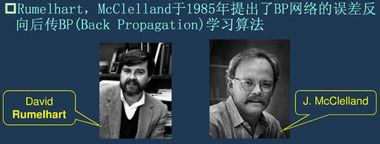BP算法是一种计算偏导数的有效方法，它的基本原理是：利用前向传播最后输出的结果来计算误差的偏导数，再用这个偏导数和前面的隐藏层进行加权求和，如此一层一层的向后传下去，直到输入层(不计算输入层)，最后利用每个节点求出的偏导数来更新权重。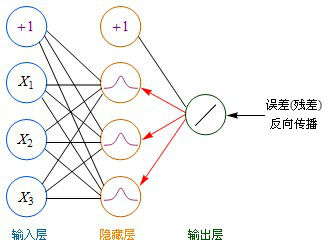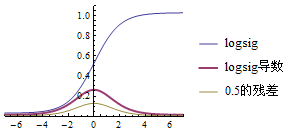BP神经网络的特点和局限：
- BP神经网络可以用作分类、聚类、预测等。需要有一定量的历史数据，通过历史数据的训练，网络可以学习到数据中隐含的知识。在你的问题中，首先要找到某些问题的一些特征，以及对应的评价数据，用这些数据来训练神经网络。
- BP神经网络主要是在实践的基础上逐步完善起来的系统，并不完全是建立在仿生学上的。从这个角度讲，实用性 > 生理相似性。
- BP神经网络中的某些算法，例如如何选择初始值、如何确定隐藏层的节点个数、使用何种激活函数等问题，并没有确凿的理论依据，只有一些根据实践经验总结出的有效方法或经验公式。
- BP神经网络虽然是一种非常有效的计算方法，但它也以计算超复杂、计算速度超慢、容易陷入局部最优解等多项弱点著称，因此人们提出了大量有效的改进方案，一些新的神经网络形式也层出不穷。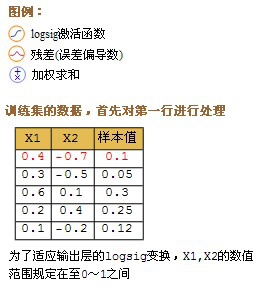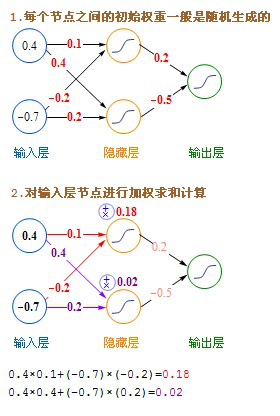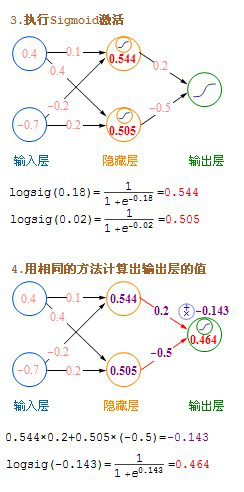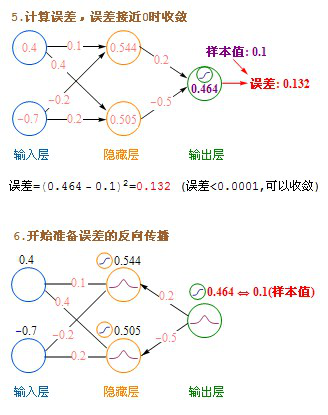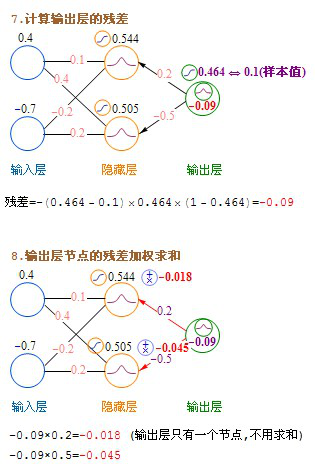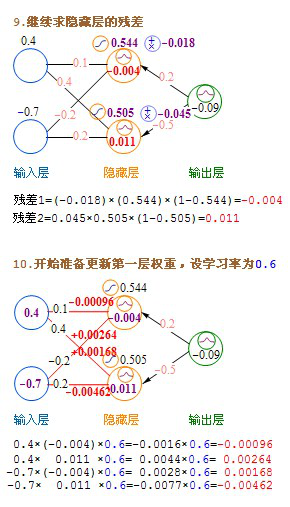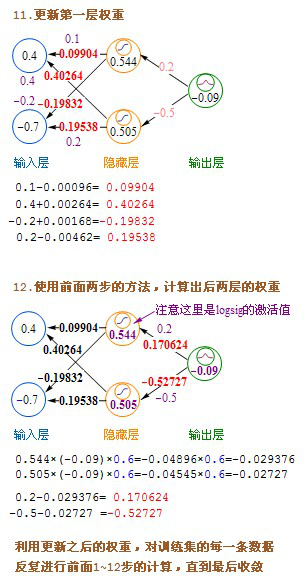Andrew Ng的《机器学习》公开课: https://class.coursera.org/ml
Coursera公开课笔记中文版（神经网络的表示）: http://52opencourse.com/139/coursera公开课笔记-斯坦福大学机器学习第八课-神经网络的表示-neural-networks-representation
Coursera公开课视频（神经网络的学习）: http://52opencourse.com/289/coursera公开课视频-斯坦福大学机器学习第九课-神经网络的学习-neural-networks-learning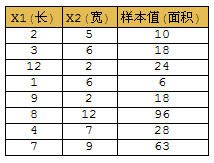p = [2 5; 3 6; 12 2; 1 6; 9 2; 8 12; 4 7; 7 9]’; % 特征数据X1,X2
t = [10 18 24 6 18 96 28 63]; % 样本值
net = newff(p, t, 20); % 创建一个BP神经网络 ff=FeedForward
net = train(net, p, t); % 用p,t数据来训练这个网络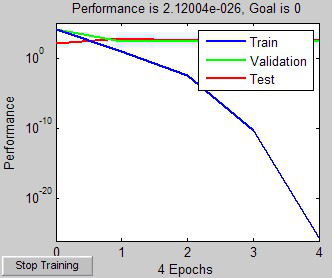s = [3 7; 6 9; 4 5; 5 7]’; % 准备一组新的数据用于测试
y = sim(net, s) % 模拟一下，看看效果
% 结果是：25.1029 61.5882 29.5848 37.5879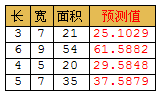p = rand(1,50)*7 % 生成1行50个0~7之间的随机数
t = sin(p) % 计算正弦曲线
s = [0:0.1:7]; % 生成0~7的一组数据，间隔0.1，用于模拟测试
plot(p, t, ‘x’) % 画散点图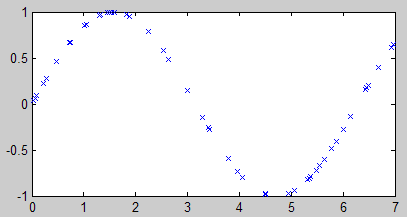net = newff(p, t, 20); % 创建神经网络
net = train(net, p, t); % 开始训练

y = sim(net, s); % 模拟
plot(s, y, ‘x’) % 画散点图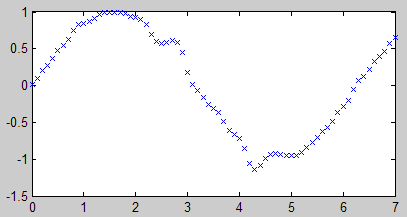% 创建3层神经网络 [隐藏层10个节点->logsig, 输出层1个节点->purelin] traingd代表梯度下降法
net = newff(p, t, 10, {‘logsig’ ‘purelin’}, ‘traingd’); % 10不能写成[10 1]

% 设置训练参数
net.trainparam.show = 50; % 显示训练结果(训练50次显示一次)
net.trainparam.epochs = 500; % 总训练次数
net.trainparam.goal = 0.01; % 训练目标：误差<0.01
net.trainParam.lr = 0.01; % 学习率(learning rate)

net = train(net, p, t); % 开始训练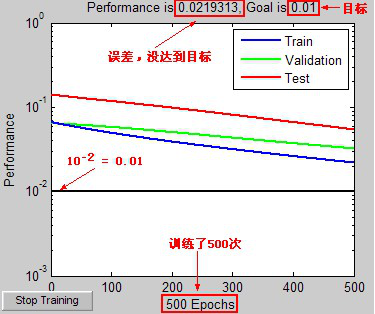y = sim(net, s); % 模拟
plot(s, y, ‘x’) % 画散点图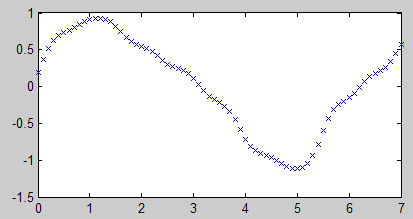% 创建2层神经网络 [隐藏层10个节点->logsig, 输出层1个节点->purelin] traingd代表梯度下降法
net = newff(p, t, 10, {‘logsig’ ‘purelin’}, ‘traingd’);

% 设置训练参数
net.trainparam.show = 50; % 每间隔50次显示一次训练结果
net.trainparam.epochs = 9999; % 总训练次数
net.trainparam.goal = 0.001; % 训练目标：误差<0.001
net.trainParam.lr = 0.06; % 学习率(learning rate)

net = train(net, p, t); % 开始训练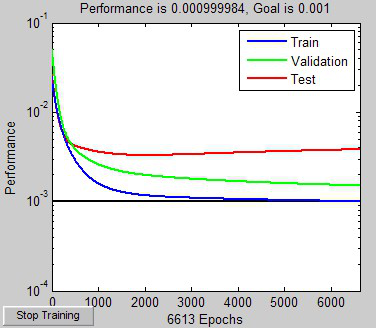y = sim(net, s); % 模拟
plot(s, y, ‘x’) % 画散点图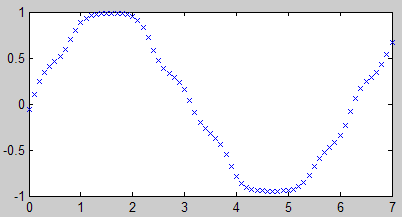net = newff(p, t, 10, {‘logsig’ ‘purelin’}, ‘trainlm’);
… 后面的代码不变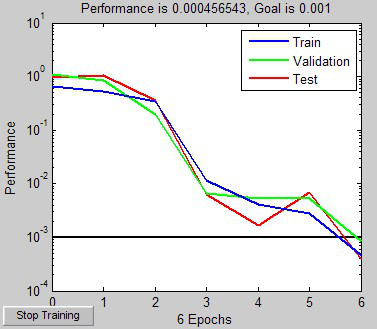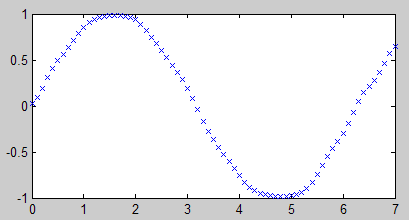net = newff(p, t, 3, {‘logsig’ ‘purelin’}, ‘trainlm’);
… 后面的代码不变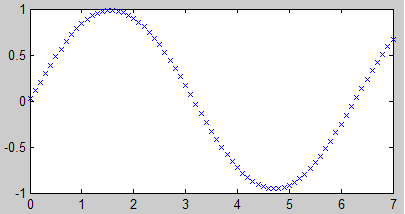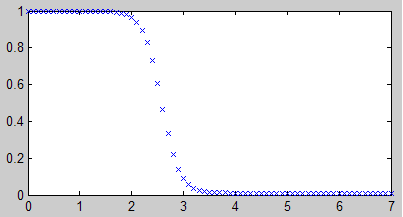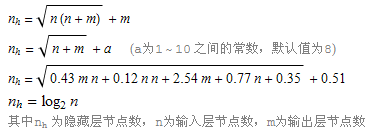net = newff(p, t, 3, {‘logsig’ ‘logsig’}); % 创建神经网络
net = train(net, p, t); % 开始训练
y = sim(net, s); % 模拟
plot(s, y, ‘x’) % 画散点图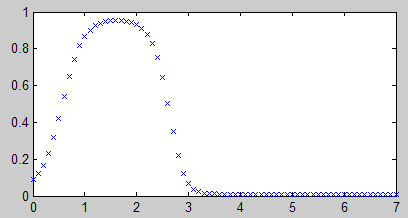0.1~0.9的范围：(0.9-0.1)(x-min)/(max-min)(0.9-0.1)+0.1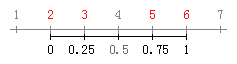Matlab的归一化命令为：mapminmax

a = [5, 2, 6, 3];

b = mapminmax(a, 0, 1) % 归一化到0~1之间
% b = 0.7500 0 1.0000 0.2500

c = mapminmax(a) % 归一化到-1~1之间
% c = 0.5000 -1.0000 1.0000 -0.5000

a = [5, 2, 6, 3];
[c,PS] = mapminmax(a); % PS记录归一化时的比例
mapminmax(‘reverse’, c, PS) % 利用PS反归一化
% ans = 5 2 6 3

p = rand(1,50)*7; % 特征数据
t = sin(p); % 样本值
s = [0:0.1:7]; % 测试数据

[pn, ps] = mapminmax(p, 0, 1); % 特征数据归一化
[tn, ts] = mapminmax(t, 0, 1); % 样本值归一化
sn = mapminmax(‘apply’, s, ps); % 测试数据，按ps比例缩放

net = newff(pn, tn, [5 1], {‘logsig’ ‘logsig’}); % 创建神经网络
net = train(net, pn, tn); % 开始训练

yn = sim(net, sn); % 模拟
y = mapminmax(‘reverse’, yn, ts); % 按ps的比例还原
plot(s, y, ‘x’) % 画散点图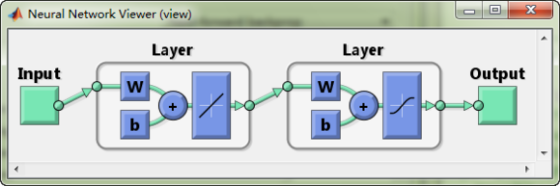PS: 这里的公式我都给出了求解过程，但如今这个年头，用手工解题的人越来越少了，一般的方程用软件来解就行了。

dsolve(‘Dx=x*(1-x)’)
% ans = 1/(1+exp(-t)*C1)

logsig

Sigmoid函数(S形函数，Logistic Function)是受统计学模型的启发而产生的激活函数。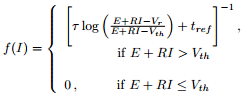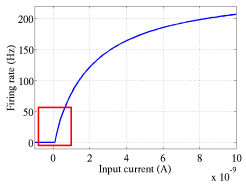Sigmoid函数原先是个描述人口增长的数学模型，1838提出，给出的是导数形式(概率密度)。人口增长规律：起初阶段大致是指数增长；然后逐渐开始变得饱和，增长变慢；达到成熟时几乎停止增长；整个过程形如一条S型曲线。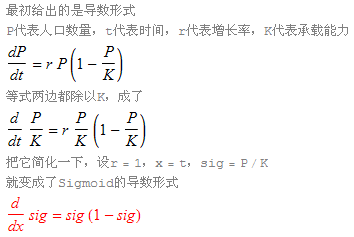Matlab可以用quiver命令来画斜率场。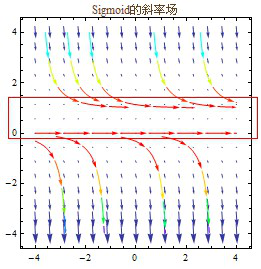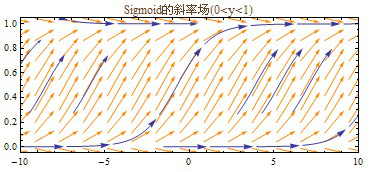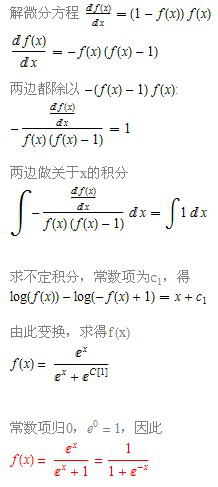tansig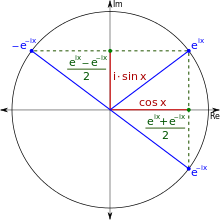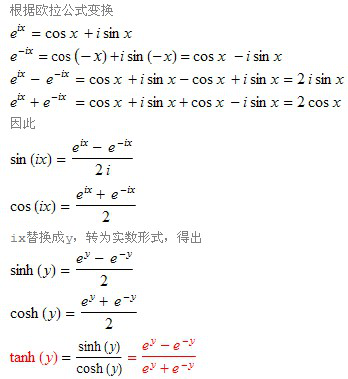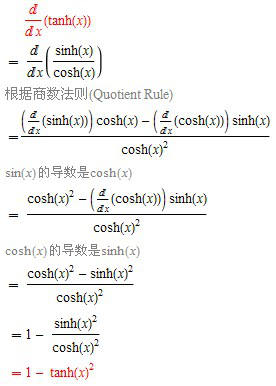logsig和tansig的关系：